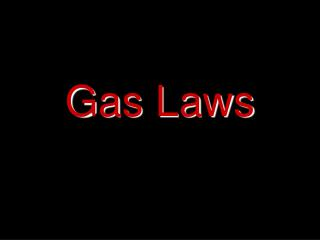Download PresentationGas Laws

# Gas Laws - PowerPoint PPT PresentationDownload Presentation## Gas Laws

- - - - - - - - - - - - - - - - - - - - - - - - - - - E N D - - - - - - - - - - - - - - - - - - - - - - - - - - -
##### Presentation Transcript

1. Gas Laws

2. Properties of Gases • Particles far apart • Particles move freely • Indefinite shape • Indefinite volume • Easily compressed • Motion of particles is constant and random

3. Gas Pressure • Gas pressure is the result of collisions of particles with their container. • More collisions = more pressure • Less collisions = less pressure • Unit = kPa or atm (also torr or mmHg)

4. Units of Pressure • 1 atm = 101.3 kPa =760 torr = 760 mmHg • 1 atm = 101,325 Pa • 1 atm = 14.70 lb/in2 • 1 bar = 100,000 Pa = 0.9869 atm atm = atmosphere

5. Amount of Gas • If you add gas, then you increase the number of particles • This will increase the number of collisions… increasing gas pressure • Unit = mole

6. Volume • Decreasing the volume of a container results in more collisions with the side of the container and therefore an increase in gas pressure • Unit = L

7. Temperature • If the temp. of a gas increases, then the kinetic energy of the particles increase. • Increasing KE makes the particles move faster. • Faster moving particles hit the sides of the container more and increase gas pressure. • Unit = Kelvin (K) (K = °C + 273)

8. STP • Standard Temperature and Pressure Standard Temp = 273K Standard Pressure = 1 atm (101.3kPa, 760torr, 760mmHg)

9. Drill • No drill today • But… • It’s March Madness • So • Move back one seat

10. Objectives • iWBAT • solve Charles, Gay-Lussac, Combined and Ideal gas law problems.

11. Gas Laws • Boyle’s Law • Charles’s Law • Gay-Lussac’s Law • Avogadro’s Law • Combined Gas Law • Ideal Gas Law • Dalton’s Law of Partial Pressures

12. Boyle’s Law • As pressure of a gas increases, the volume decreases (if the temp is constant). • Inverse relationship P1V 1= P2V2

13. Charles’s Law • As temperature of a gas increases, the volume increases (if pressure is constant). • Direct relationship V 1= V2 T 1 T2

14. Gay-Lussac’s Law • As temperature of a gas increases, the pressure increases (if volume is constant). • Direct relationship P 1 = P2 T 1 T2

15. Combined Gas Law P1V1 = P2V2 T 1 T2

16. Avogadro’s Law • Equal volumes of gases at the same temperature and pressure contain an equal number of particles V 1 = V2 n 1 n2

17. Dalton’s Law of Partial Pressure • The sum of the partial pressures of all the components in a gas mixture is equal to the total pressure of the gas in a mixture. • In other words…all the individual pressures in a gas mixture add up to the total pressure. Ptotal = P1 + P2 + P3 + …

18. Dalton’s Law of Partial Pressure is used whenever a gas is obtained over water because there will also be water vapor present. • You will have to look up the pressure of water at a given temperature.

19. Homework • Pg 367 #1-5 (use Table A8 on pg 859 for #5) • Pg 375 #1-6 (use the Kinetic Molecular Theory assumption #2 and #5 on pg. 329 for #1 & #6 respectively)

20. Ideal Gas Law • The most important gas law!

21. Ideal Gas Law • An Ideal Gas does not exist, but the concept is used to model gas behavior • A Real Gas exists, has intermolecular forces and particle volume, and can change states.

22. Ideal Gas Law PV = nRT P = Pressure (atm) V = Volume (L) n = # of particles (mol) T = Temperature (K) R = Ideal gas constant 8.31 (kPa∙L) or 0.0821 (atm∙L) (mol∙K) (mol∙K)

23. At what temperature would 4.0 moles of hydrogen gas in a 100 liter container exert a pressure of 1.00 atm? Ideal Gas Law PV = nRT T = PV/nR = (1.00atm)(100L) (4.0mol)(.0821atm∙L/mol∙K) = 304.5 K  300K Use Ideal Gas Law when you don’t have more than one of any variable

24. Extensions! • PV = nRT n is moles. If we know the chemical formula for the gas we can convert moles to mass or to particles using Dimensional Analysis!

25. We could also use the fact that: moles = mass or n = m molar mass MM Plugging this in, we have PV = mRT MM This can be rearranged to solve for Density which is m/V m = P∙MM or D = P∙MM V R∙T R∙T

26. What is the density of water vapor at STP? D = P∙MM D = (1 atm)(18.02g/mol) R∙T (.0821atm∙L)(273K) mol∙K D = 0.804 g/L

27. Practice • Try problems # 5 & 6 on the Ideal Gas Law WS

28. Solve it! • What volume does 0.0881 moles of gas occupy at 110.4 kPa and 29°C?

29. Problem #1 • Oxygen occupies a volume of 66L at 6.0atm. What volume will it occupy at 920kPa?

30. Problem #2 • At 25°C a gas has a volume of 6.5mL. What volume will the gas have at 50.°C?

31. Problem #3 Initially you have gas at 640mmHg, 2.5L, and 22°C. What is the new temperature at 750mmHg and 5L?